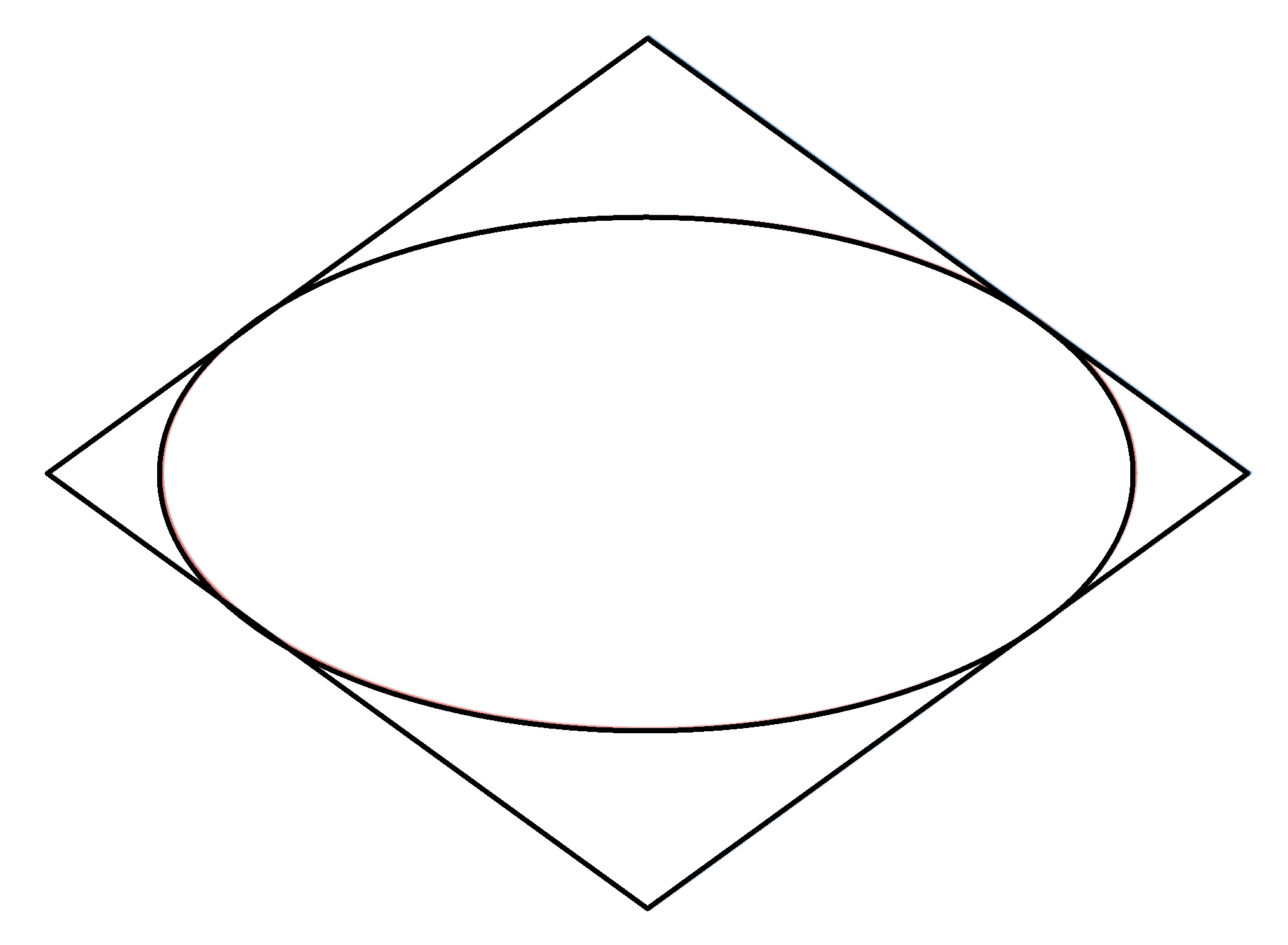# Ellipse's Circumscribed PerimeterAround an ellipse of eccentricity $e = 0.85$ circumscribe a quadrilateral with minimum perimeter and vertices on the major and minor axes of the ellipse.

If the area of the ellipse is $A_1$ and the area of the quadrilateral is $A_2$, find $\dfrac{A_1}{A_2}\times 100$.

Try similar problems

All of my problems are original.

×